• +91 9971497814
• info@interviewmaterial.com

# Chapter 15- Waves Interview Questions Answers

### Related Subjects

Question 1 : A string of mass 2.50 kg is under a tension of 200 N. The length of the stretched string is 20.0 m. If the transverse jerk is struck at one end of the string, how long does the disturbance take to reach the other end?

Mass of the string, M = 2.50 kg
Tension in the string, T = 200 N
Length of the string, l = 20.0 m
Mass per unit length,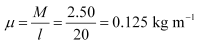The velocity (v) of the transverse wave in the string is given by the relation: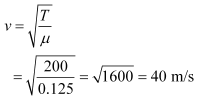∴Time taken by the disturbance to reach the other end, t =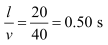Question 2 : A stone dropped from the top of a tower of height 300 m high splashes into the water of a pond near the base of the tower. When is the splash heard at the top given that the speed of sound in air is 340 m s–1? (g= 9.8 m s–2)

Height of the tower, s = 300 m
Initial velocity of the stone, u = 0
Acceleration, a = g = 9.8 m/s2
Speed of sound in air = 340 m/s
The time (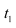) taken by the stone to strike the water in the pond can be calculated using the second equation of motion, as: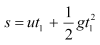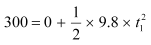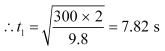Time taken by the sound to reach the top of the tower,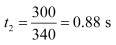Therefore, the time after which the splash is heard,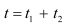= 7.82 + 0.88 = 8.7 s

Question 3 : A steel wire has a length of 12.0 m and a mass of 2.10 kg. What should be the tension in the wire so that speed of a transverse wave on the wire equals the speed of sound in dry air at 20 °C = 343 m s–1.

Length of the steel wire, l =12 m

Mass of the steel wire, m =2.10 kg

Velocity of the transverse wave, v =343 m/s

Mass per unit length,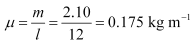For tension T, velocity of thetransverse wave can be obtained using the relation: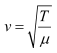T = v2 µ

= (343)2 × 0.175 =20588.575 ≈ 2.06 × 104 N

Question 4 :
Use the formula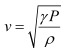to explain why the speed of sound in air
(a) is independent of pressure,
(b) increases with temperature,
(c) increases with humidity.

(a) Take the relation: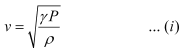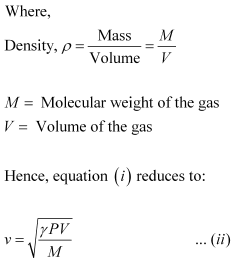Now from the ideal gas equation for n = 1:

PV = RT

For constant T, PV = Constant

Since both M and γ are constants, v = Constant

Hence, at a constant temperature, the speed of sound in a gaseous medium is independent of the change in the pressure of the gas.

(b) Take the relation:For one mole of an ideal gas, the gas equation can be written as:
PV = RT
P =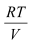… (ii)
Substituting equation (ii) in equation (i), we get: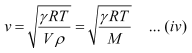Where,
Mass, M = ρV is a constant
γ and R are also constants
We conclude from equation (iv) that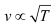.
Hence, the speed of sound in a gas is directly proportional to the square root of the temperature of the gaseous medium, i.e., the speed of the sound increases with an increase in the temperature of the gaseous medium and vice versa.
(c) Let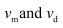be the speeds of sound in moist air and dry air respectively.
Let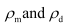be the densities of moist air and dry air respectively.
Take the relation:Hence, the speed of sound in moist air is: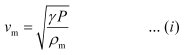And the speed of sound in dry air is: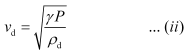On dividing equations (i) and (ii), we get: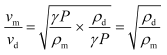However, the presence of water vapour reduces the density of air, i.e.,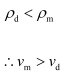Hence, the speed of sound in moist air is greater than it is in dry air. Thus, in a gaseous medium, the speed of sound increases with humidity.

Question 5 :
You have learnt that a travelling wave in one dimension is represented by a function y = f (x, t)where x and t must appear in the combination x – v t or x + v t, i.e. y = f (x ± v t). Is the converse true? Examine if the following functions for y can possibly represent a travelling wave:
(a) (x – vt)2
(b)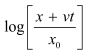(c)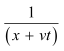(a) Does not represent a wave
(b) Represents a wave
(c) Does not represent a wave
The converse of the given statement is not true. The essential requirement for a function to represent a travelling wave is that it should remain finite for all values of x and t.
Explanation:
(a) For x = 0 and t = 0, the function (x – vt)2  becomes 0.
Hence, for x = 0 and t = 0, the function represents a point and not a wave.
(b) For x = 0 and t = 0, the function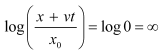Since the function does not converge to a finite value for x = 0 and t = 0, it represents a travelling wave.
(c) For x = 0 and t = 0, the function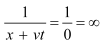Since the function does not converge to a finite value for x = 0 and t = 0, it does not represent a travelling wave.

Question 6 : A bat emits ultrasonic sound of frequency 1000 kHz in air. If the sound meets a water surface, what is the wavelength of (a) the reflected sound, (b) the transmitted sound? Speed of sound in air is 340 m s–1 and in water 1486 m s–1.

(a) Frequency of the ultrasonic sound, ν = 1000 kHz = 106 Hz

Speed of sound in air, va =340 m/s

The wavelength (λr) ofthe reflected sound is given by the relation: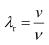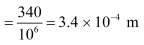(b) Frequency of the ultrasonic sound, ν = 1000 kHz = 106 Hz

Speed of sound in water, vw =1486 m/s

The wavelength of the transmitted sound is given as: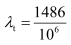=1.49 × 10–3 m

Question 7 :

A hospital uses an ultrasonic scanner tolocate tumours in a tissue. What is the wavelength of sound in the tissue inwhich the speed of sound is 1.7 km s–1? The operatingfrequency of the scanner is 4.2 MHz.

Speed of sound in the tissue, v =1.7 km/s = 1.7 × 103 m/s

Operating frequency of the scanner, ν = 4.2MHz = 4.2 × 106 Hz

The wavelength of sound in the tissue is given as: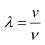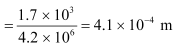Question 8 :
A transverse harmonic wave on a string is described by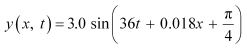Where x and y are in cm and t in s. The positive direction of x is from left to right.
(a) Is this a travelling wave or a stationary wave?
If it is travelling, what are the speed and direction of its propagation?
(b) What are its amplitude and frequency?
(c) What is the initial phase at the origin?
(d) What is the least distance between two successive crests in the wave?

(a) Yes; Speed = 20 m/s, Direction = Right to left

(b) 3 cm; 5.73 Hz

(c)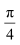(d) 3.49 m

Explanation:

(a) The equation of a progressive wave travelling from rightto left is given by the displacement function:

y (xt)= a sin (ωt + kx + Φ) … (i)

The given equation is: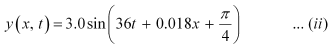On comparing both the equations, we findthat equation (ii) represents a travelling wave, propagating from rightto left.

Now, using equations (i) and (ii),we can write:

ω = 36 rad/s and k = 0.018m–1

We know that: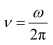and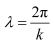Also,

v = νλ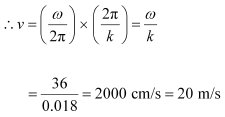Hence, the speed of the given travelling wave is 20 m/s.

(b) Amplitude of the given wave, a = 3 cm

Frequency of the given wave: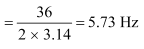(c) On comparing equations (i) and (ii), we findthat the initial phase angle,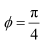(d) The distance between two successive crests or troughs isequal to the wavelength of the wave.

Wavelength is given by the relation: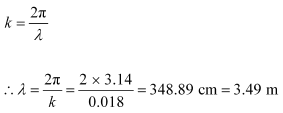Question 9 : For the wave described in Exercise 15.8, plot the displacement (y) versus (t) graphs for x = 0, 2 and 4 cm. What are the shapes of these graphs? In which aspects does the oscillatory motion in travelling wave differ from one point to another: amplitude, frequency or phase?

All the waves have different phases.
The given transverse harmonic wave is: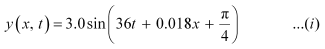For x = 0, the equation reduces to: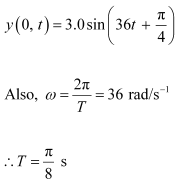Now, plotting y vs. t graphsusing the different values of t, as listed in the given table.

 t (s) 0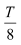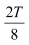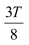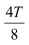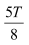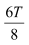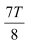y (cm)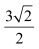30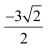–30

For x = 0, x =2, and x = 4, the phases of the three waves will get changed.This is because amplitude and frequency are invariant for any change in x.The yt plots of the three waves are shown in the givenfigure.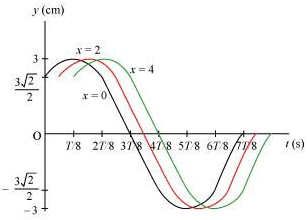Question 10 :
For the travelling harmonic wave
y (x, t) = 2.0 cos 2π (10t – 0.0080x + 0.35)
Where x and y are in cm and t in s. Calculate the phase difference between oscillatory motion of two points separated by a distance of
(a) 4 m,
(b) 0.5 m,
(c)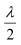,
(d)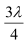Equation for a travelling harmonic wave is given as:

y (xt)= 2.0 cos 2π (10t – 0.0080x + 0.35)

= 2.0 cos (20πt – 0.016πx +0.70 π)

Where,

Propagation constant, k =0.0160 π

Amplitude, = 2 cm

Angular frequency, ω= 20 π rad/s

Phase difference is given by the relation: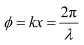(a) For x = 4 m = 400 cm

Φ = 0.016 π × 400 = 6.4 π rad

(b) For 0.5 m = 50 cm

Φ = 0.016 π × 50 = 0.8 π rad

(c) For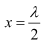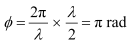(d) For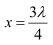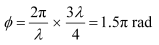Todays Deals### Chapter 15- Waves Contributorskrishan

Name:
Email:

# Latest News# 9000 interview questions in different categories